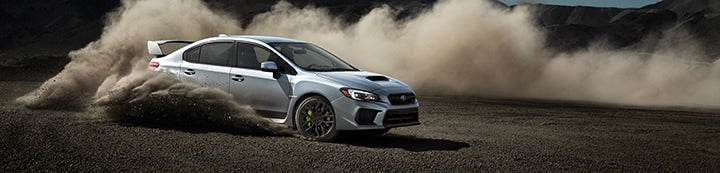1 - 6 of 6 Posts

#### DTsRex

·
##### Registered
Joined
·
89 Posts
Discussion Starter · ·
My 2002 WRX sedan was dyno'ed at 247 hp at the tire and 217 torque. What does the torque translate to at the crank?

I believe there's about a 60hp loss from the crank to the tires, but I don't know what it is for torque. Thanks.

#### 0db

·
##### Registered
Joined
·
139 Posts
Dynos really only measure torque - horsepower is calculated from that. Since they're mathematically dependent on each other, the factor you use to correct HP from wheel to crank is the exact same one you would use for torque.

For example, if you have some reason to believe that there is 20% driveline loss between flywheel and drive wheels, and you dyno at 300 hp and 200 lb.ft. of torque, multiply both values by 1.20 to yield your approximate flywheel numbers of 360 hp and 240 lb.ft. torque.

#### Felix

·
##### Registered
Joined
·
9 Posts
0db said:
Dynos really only measure torque - horsepower is calculated from that. Since they're mathematically dependent on each other, the factor you use to correct HP from wheel to crank is the exact same one you would use for torque.

For example, if you have some reason to believe that there is 20% driveline loss between flywheel and drive wheels, and you dyno at 300 hp and 200 lb.ft. of torque, multiply both values by 1.20 to yield your approximate flywheel numbers of 360 hp and 240 lb.ft. torque.
I know Im knew, but your math is incorrect if it's actually a 20% drivetrain loss. When reading this post, I decided to setup the numbers in a proportion.

flywheel horsepower _ wheel horsepower
----------------------- _ ------------------------
100% of actual power 80% of actual power (whp)

Take your wheel horsepower (or torque) and multiply it by 100, then divide by 80.

#### 0db

·
##### Registered
Joined
·
139 PostsThat's what I get for not thinking before I post.

You're absolutely correct mathematically. If you've got a 20% loss from crank to wheel, you would multiply the whp and wtq numbers by 100 / (100 - 20), which ends up being 1.25

Thanks!

(my point stands about the correction factor equally affecting hp and torque numbers)

#### dark_rex

·
##### Banned
Joined
·
7,636 Posts
what type of dyno did you dyno on?

if you know that, you can figure the number out.

dR

#### 0db

·
##### Registered
Joined
·
139 Posts
dark_rex said:
what type of dyno did you dyno on?

if you know that, you can figure the number out.How does the type of dyno factor into a flywheel-wheel power conversion? Even within brands of dyno, the final output is dependent on the calibration and correction factors fed into the dyno in the first place.

1 - 6 of 6 Posts